# Chapter 6 Factorisation of Algebraic Expressions RD Sharma Solutions Exercise 6.5 Class 9 Maths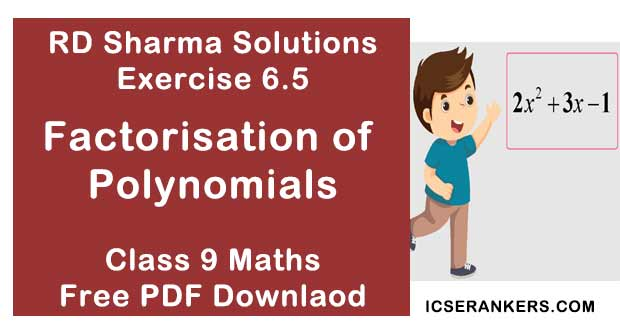Chapter Name RD Sharma Chapter 6 Factorisation of Polynomials Exercise 6.5 Book Name RD Sharma Mathematics for Class 10 Other Exercises Exercise 6.1Exercise 6.2Exercise 6.3Exercise 6.4 Related Study NCERT Solutions for Class 10 Maths

### Exercise 6.5 Solutions

Using factor theorem, factorize each of the following polynomials :

1. x3 + 6x2 + 11x + 6

Solution

Let f(x) = x3 + 6x2 + 11x + 6 be the given polynomial.
The constant term in f(x) is 6 and factors of 6 are ±1, ±2, ±3 and ±6
Putting x = -1 in f(x), we have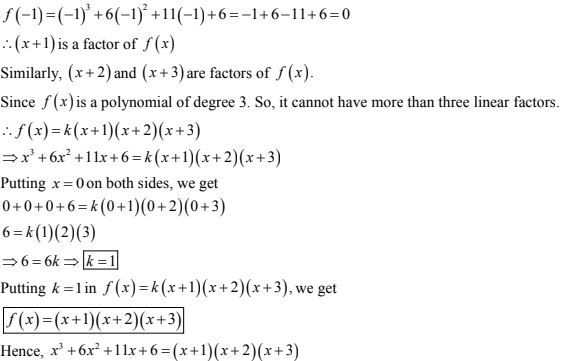2. x3 + 2x2 - x - 2

Solution

Let f(x) = x3 + 2x2 - x - 2
The constant term in f(x) is equal to -2 and factors of -2 and ±1, ±2
Putting x = 1 in f(x) , we have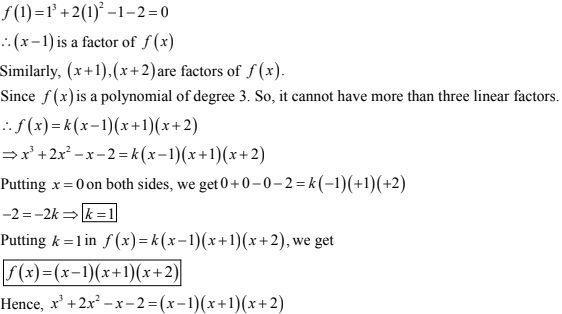3. x3 - 6x2 + 3x + 10

Solution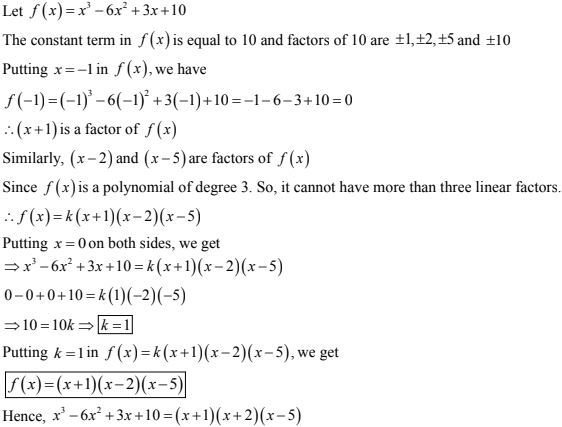4. x4 - 7x3 + 9x2 + 7x - 10

Solution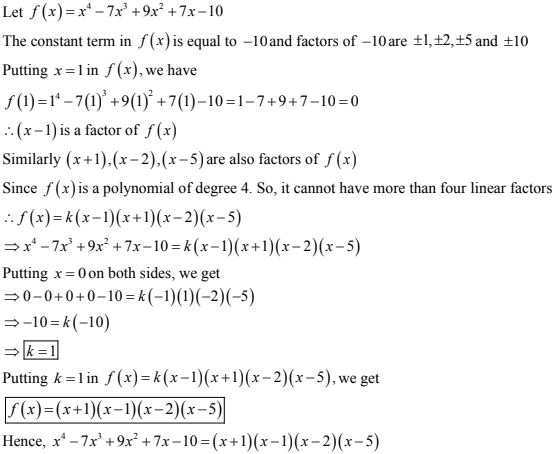5. x4 -2x3 - 7x2 + 8x + 12

Solution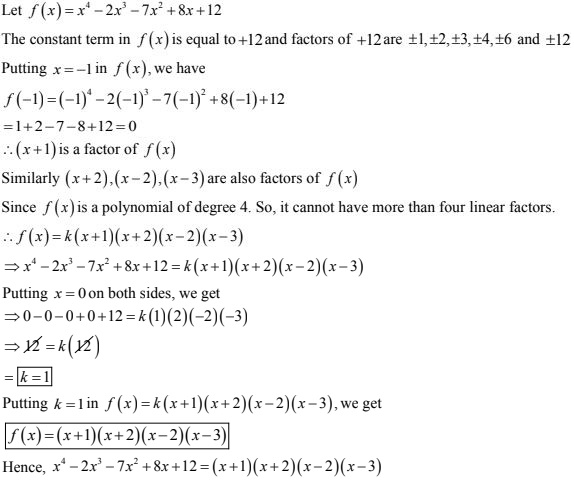6. x4 + 10x3 + 35x2 + 50x + 24

Solution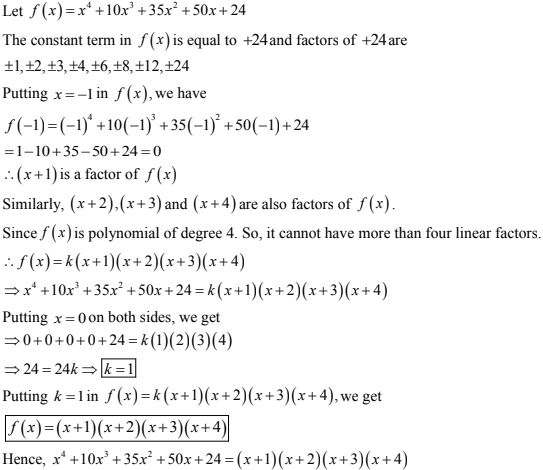7. 2x4 - 7x3 - 13x2 + 63x - 45

Solution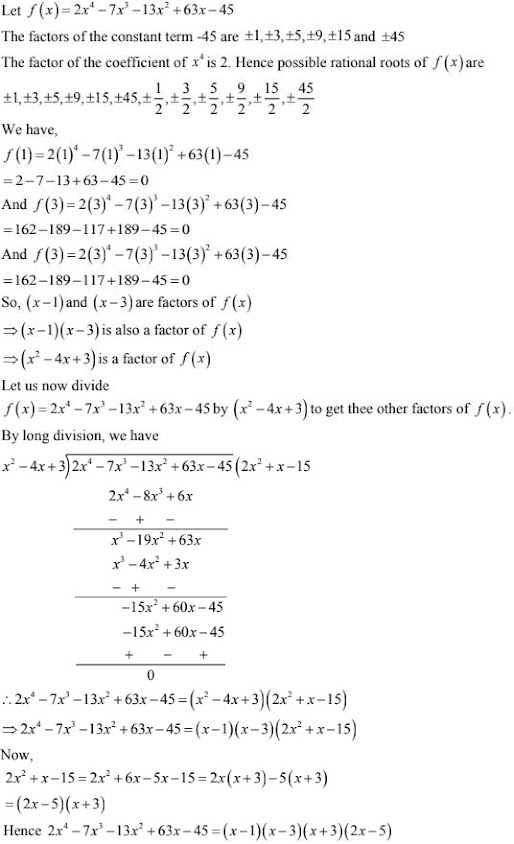8. 3x3 - x3 - 3x + 1

Solution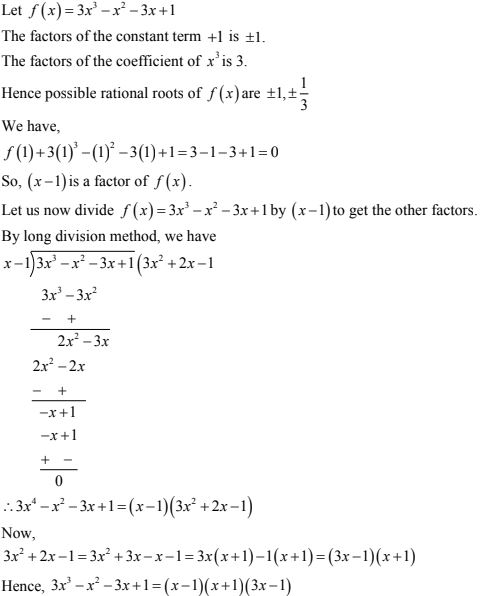9. x3 - 23x2 + 142x - 120

Solution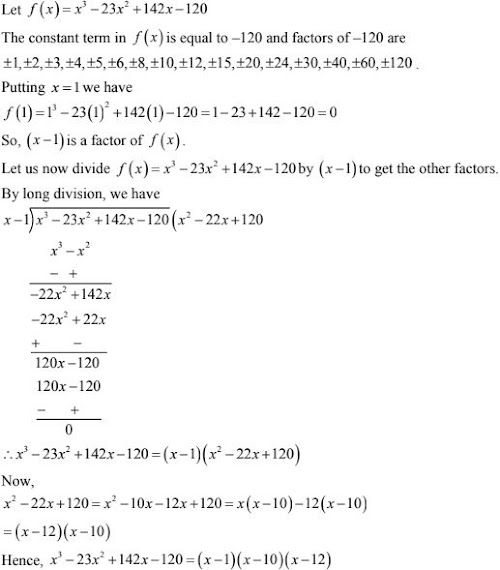10. y3 - 7y + 6

Solution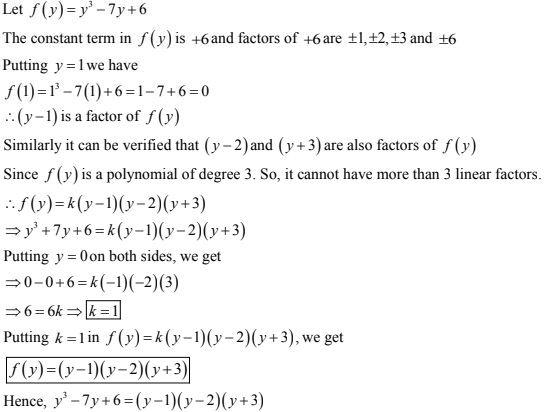11. x3 - 10x2 - 53x - 42

Solution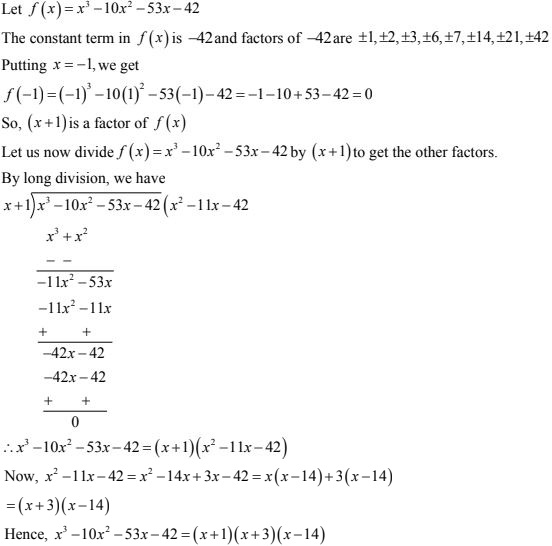12. y3 - 2y2 - 29y - 42

Solution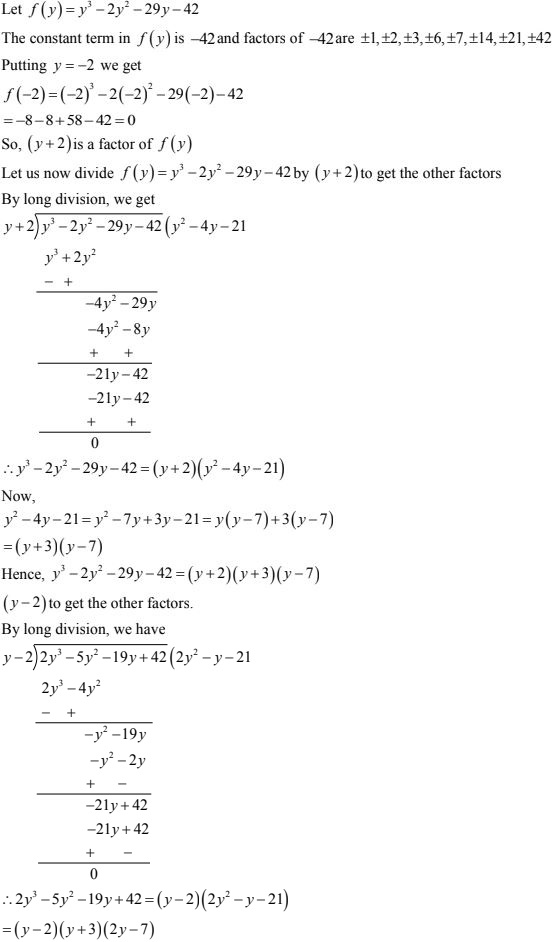13. 2y3 - 5y2 - 19y + 42

Solution

(y - 2)(y + 3)(2y - 7)

14. x3 + 13x2 + 32x + 20

Solution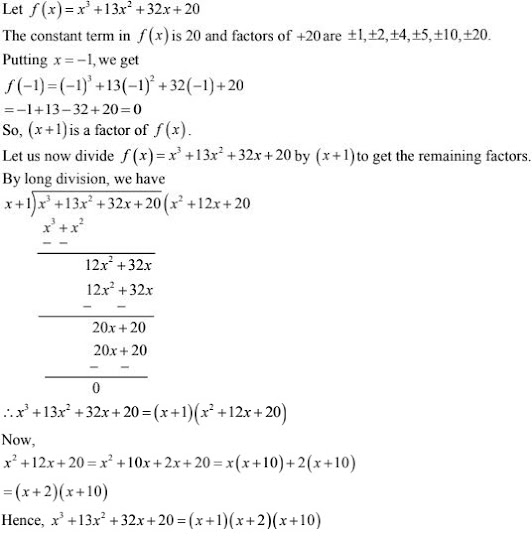15. x3 - 3x2 - 9x - 5

Solution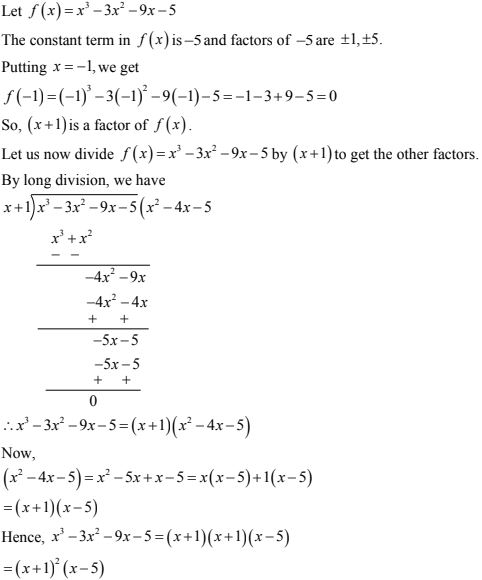16. 2y3 + y2 - 2y - 1

Solution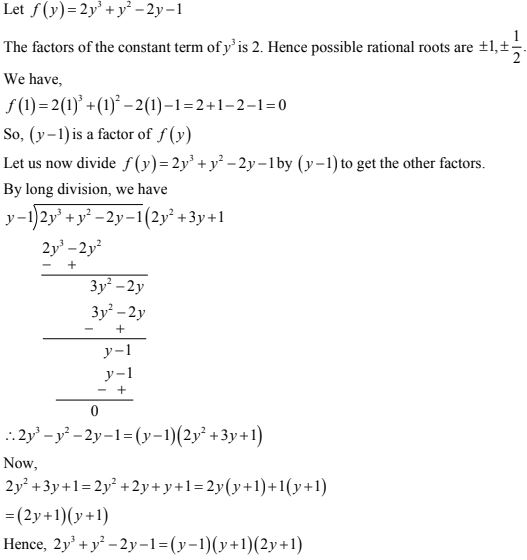17. x3 - 2x2 - x + 2

Solution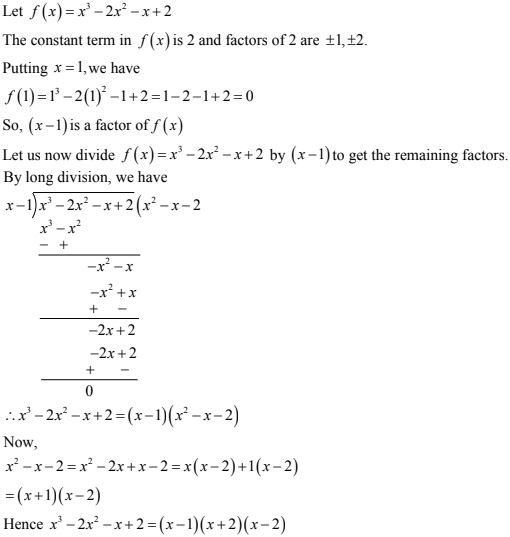18. Factorize each of the following polynomials:
(i) x3 + 13x2 + 31x - 45 given that x + 9 is a factor
(ii) 4x3 + 20x2 + 33x + 18 given that 2x + 3 is a factor

Solution